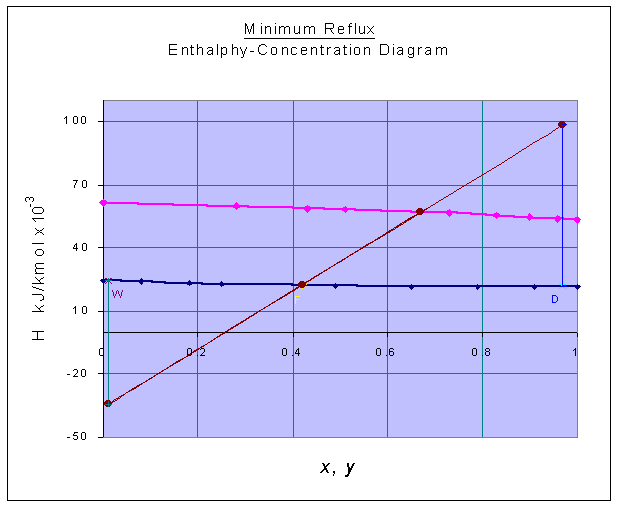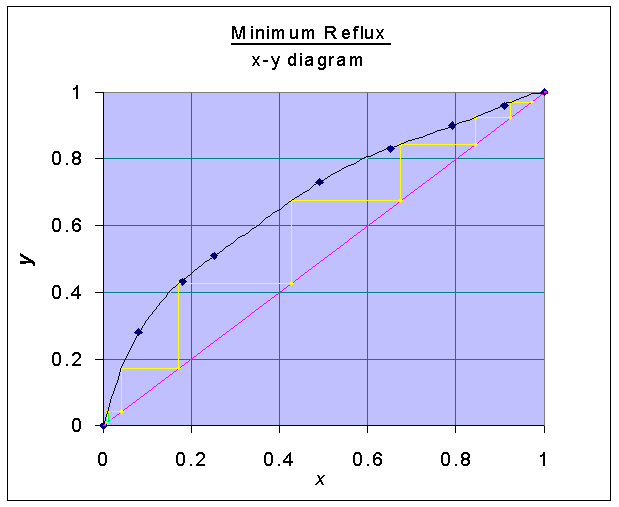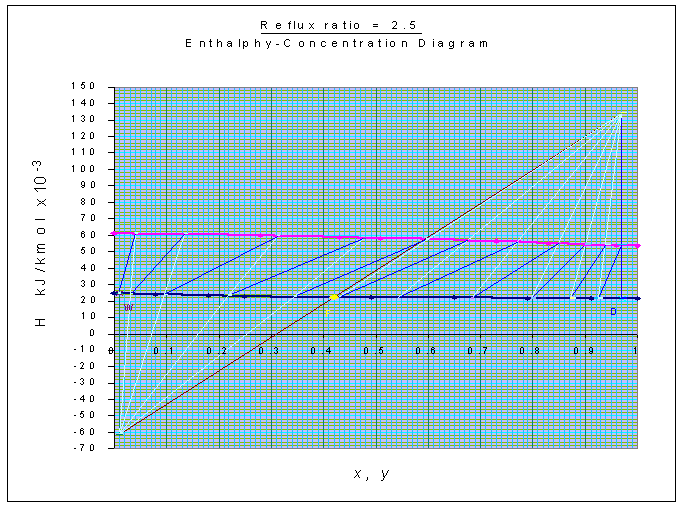### Ponchon-Savarit Method

1000 kg/hr of a mixture containing 42 mole percent heptane and 58 mole percent ethyl benzene is to be fractionated to a distillate containing 97 mole percent heptane and a residue containing 99 mole percent ethyl benzene using a total condenser and feed at its saturated liquid condition. The enthalpy-concentration data for the heptane-ethyl benzene at 1 atm pressure are as follows:

 xheptane 0 0.08 0.18 0.25 0.49 0.65 0.79 0.91 1 yheptane 0 0.28 0.43 0.51 0.73 0.83 0.9 0.96 1 Hl (kJ/kmol) x 10-3 24.3 24.1 23.2 22.8 22.05 21.75 21.7 21.6 21.4 Hv (kJ/kmol) x 10-3 61.2 59.6 58.5 58.1 56.5 55.2 54.4 53.8 53.3

Calculate the following:

1. Minimum reflux ratio
2. Minimum number of stages at total reflux
3. Number of stages at reflux ratio of 2.5
4. Condenser duty
5. Reboiler duty

Calculations:

Heptane = C7H16

Ethyl benzene = C6H5C2H5

Average molecular weight of feed solution

= 0.42 x 100 + 0.58 x 106 = 103.48

Molal flow rate of feed, F = 1000/103.48 = 9.9937 kmol/hr

The H-x-y diagram is constructed with the above data as given below:zF = 0.42

HF = 22.30098 x 103 kJ/kmol (from graph)

xD = 0.97

HD = 21.53695 x 103 kJ/kmol (from graph)

xW = 0.01

HW = 24.27584 x 103 kJ/kmol (from graph)

At minimum reflux ratio, the tie-line passing through F determines Q' and Q"

Q' = 98.4391 x 103 kJ/kmol (from graph)

Q" = -34.4537 x 103 kJ/kmol (from graph)

HG1 = 53.70453 x 103 kJ/kmol (from graph)

HL0 = HD = 21.53695 x 103 kJ/kmol

Reflux ratio, R = (Q' - HG1) / (HG1 - HL0) = (98.4391 - 53.70453) / (53.70453 - 21.53695)

= 1.3907

Minimum Reflux ratio = 1.3907

With the x-y data, the following graph is drawn:Minimum number of stages at total reflux is found from the x-y diagram and = 6.97

Number of stages at reflux ratio of 2.5:

(Q' - 53.70453) / (53.70453 - 21.53695)= 2.5

Q' = 134.1235 x 103 kJ/kmol

F = 9.9937 kmol/hr

Material balance equations:

F = D + W

F zF = D xD + W xW

i.e.

9.9937 = D + W

9.9937 x 0.42 = 0.97 D + 0.01 W

Solving,

0.96 D = 4.0974

D = 4.2681 kmol/hr

W = 9.9937 - 4.2681 = 5.7256 kmol/hr

Energy balance equation:

F HF = D Q' + W Q"

Substituting for the known quantities,

9.9937 x 22.30098 x 103 = 4.2681 x 134.1235 x 103 + 5.7256 x Q"

Q" = -61.0562 x 103 kJ/kmol

Q' = HD + QC / D

Q" = HW - QB / W

Substituting for the known quantities in the above equations,

134.1235 x 103 = 21.53695 x 103 + QC / 4.2681

QC = 480.53 x 103 kJ/hr = 133.48 kW

-61.0562 x 103 = 24.27584 x 103 - QB / 5.7256

QB = 488.58 x 103 kJ/hr = 135.72 kW

Condenser duty = QC = 133.48 kW

Reboiler duty = QB = 135.72 kW

Number of stages is estimated from Ponchon-Savarit method as shown in the graph, and is equal to 11 (including the reboiler).Feed is to be introduced at the 7th plate, counting from the top.

For constructing tie-lines in H-x-y diagram, x-y digram is also used.